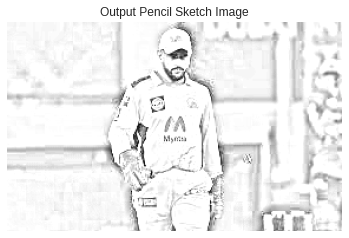# How to Convert Image into a Pencil Sketch Using Python

An image in Python is simply a two-dimensional array of integers. So, using several Python tools, one can perform a few matrix operations to obtain some quite amazing effects. To convert a regular image to a sketch, we will change its original RGB values and assign them RGB values equivalent to grey, resulting in a sketch of the input image.

Here we use OpenCV  and matplotlib modules(for plotting figures/images)

## Converting Image into a Pencil Sketch Using Python

1)Importing Modules

# Import cv2(OpenCV) module using the import keyword
import cv2
# Import pyplot of matplotlib module using the import keyword
import matplotlib.pyplot as plt
# Use the seaborn library using use() function
plt.style.use('seaborn')

2)Plotting the given Original Image

# Load some random image by passing the filepath/name of the image to the imread()
# function and store it in a variable
# Change the color code of the given image to RGB format using the cvtColor() function
# to get the original colors
gvn_image = cv2.cvtColor(gvn_image, cv2.COLOR_BGR2RGB)
# Pass some random image size to be plotted(width, length) to the figure() function
plt.figure(figsize=(6,6))
# Plot the given original image using the imshow() function
plt.imshow(gvn_image)
plt.axis("off")
# Give the Title of the image using the title() function
plt.title("Given Sample Image")
# Display the plot(image) using the plot function
plt.show()

Output: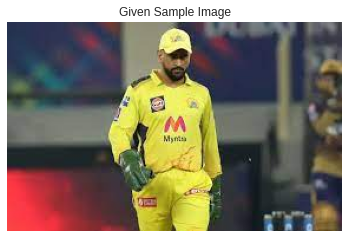3)Convert the given image to the Grayscale

The cvtColor () function to used to convert the image to a grayscale image to reduce the image’s complexity and make it easier to work with.

# Pass the given image, COLOR_BGR2GRAY as arguments to the cvtColor() function
# to convert the given image to grayscale image
# Store it in a variable
grayscle_image = cv2.cvtColor(gvn_image, cv2.COLOR_BGR2GRAY)
# Pass some random image size to be plotted(width, length) to the figure() function
plt.figure(figsize=(6,6))
# Plot the above Grayscale image for the given image using the imshow() function
plt.imshow(grayscle_image, cmap="gray")
plt.axis("off")
# Give the Title of the image using the title() function
plt.title("Grayscale Image")
# Display the grayscale image using the plot function
plt.show()

Output: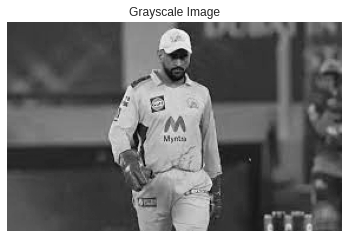4)Getting the Inverted Image

Inverting the image is the next process. Now you’re probably wondering why you’d do something like that. The reason is that inverting an image helps in the analysis of the image with greater precision and detail.

For the sketch generation, a more detailed study is necessary because the sketches must be exact and good.

# Get the inverted image of by passing the above grayscale image as an
# argument to the bitwise_not() function
invertd_image = cv2.bitwise_not(grayscle_image)
plt.figure(figsize=(6,6))
plt.imshow(invertd_image, cmap="gray")
plt.axis("off")
plt.title("Inverted Image")
plt.show()

Output: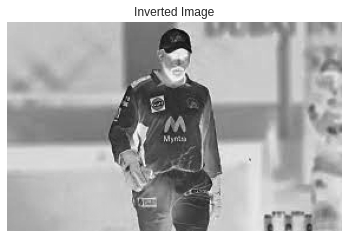5)Getting a Smoothened Image

we will smooth the image to ensure that the sketch we obtain is less edgy and smooth. The corresponding code is displayed below.

# Get the Smoothened image from the above inverted image using the
# GaussianBlur() function
smooth_image = cv2.GaussianBlur(invertd_image, (21, 21),sigmaX=0, sigmaY=0)
plt.figure(figsize=(6, 6))
plt.imshow(smooth_image, cmap="gray")
plt.axis("off")
plt.title("Smoothened Image using GaussianBlur() function")
plt.show()

Output: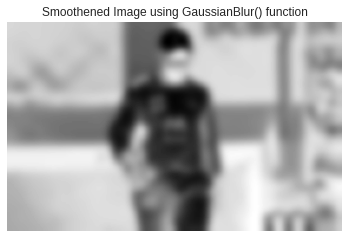6)Getting a Pencil Sketch Image

Now that we’ve completed the image processing, we’ll provide the previous outputs to the function, together with the correct and precise parameters, to make the necessary alterations to the image.

# Get the output pencil sktech image for the given image using the divide() function
# by passing the above gray scale image, smoothened image, scale as arguments to it.
pencil_skecth = cv2.divide(grayscle_image, 255 - smooth_image, scale=255)
plt.figure(figsize=(6, 6))
plt.imshow(pencil_skecth, cmap="gray")
plt.axis("off")
plt.title("Output Pencil Sketch Image")
plt.show()

Output: